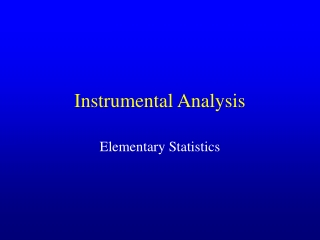DownloadDownload PresentationInstrumental Analysis

# Instrumental Analysis

Download Presentation## Instrumental Analysis

- - - - - - - - - - - - - - - - - - - - - - - - - - - E N D - - - - - - - - - - - - - - - - - - - - - - - - - - -
##### Presentation Transcript

1. Instrumental Analysis Elementary Statistics

2. • The digits in a measured quantity that are known exactly plus one uncertain digit. 29.42 mL Certain + one uncertain 29.4 mL Certain I. Significant Figures

3. Rule 1: To determine the number of significant figures in a measurement, read the number from left to right and count all digits, starting with the first non-zero digit. I. Significant Figures Rule 2: When adding or subtracting numbers, the number of decimal places in the answer should be equal to the number of decimal places in the number with the fewest places. Rule 3: In multiplication or division, the number of significant figures in the answer should be the same as that in the quantity with the fewest significant figures. Rule 4: When a number is rounded off, the last digit to be retained is increased by one only if the following digit is 5 or more.

4. II. Precision & Accuracy A. Precision – the reproducibility of a series of measurements. Poor Precision • Figures of Merit • Standard Deviation • Variance • Coefficient of Variation Good Precision

5. B. Accuracy – How close a measured value is to the known or accepted value. • Figures of Merit • Absolute Error • Percent Error (Relative Error) Good Accuracy Good Precision Poor Accuracy

6. III. Types of Error Ea = Es + Er Absolute error in a measurement arises from the sum of systematic (determinate) error and random (indeterminate) error. A. Systematic Error - has a definite value and an assignable cause. • There are three common sources of systematic error: • Instrument error • Personal error • Method error

7. B. Random Error - Uncertainty in a measurement arising from an unknown and uncontrollable source . (Also commonly referred to as Noise.) • Error fluctuates evenly in both the positive and negative direction. • Treated with statistical methods.

8. IV. Statistical Treatment of Random Error (Er) • A large number of replicate measurements result in a distribution of values which are symmetrically distributed about the mean value. A. Normal (Gaussian) Error Curve - A plot of fraction of measurements vs. the value of a measurement results in a bell shaped curve symmetrically distributed about the mean.

9. m = 50 s = 5 m = 50 s = 10 m = Population Mean (True Mean) s = Population Standard Deviation -1s +1s -1s +1s • Population refers to an infinite number of measurements A. Normal Error Curve m Relative frequency, dN / N xi

10. -1s +1s -2s +2s -3s +3s A. Normal Error Curve m • 68.3% of measurements will fall within ± s of the mean. • 95.5% of measurements will fall within ± 2s of the mean. Relative frequency, dN / N • 99.7% of measurements will fall within ± 3s of the mean. xi

11. B. Statistics • Population Mean (m) xi = individual measurement N = number of measurements

12. B. Statistics 2. Population Standard Deviation (s) xi = individual measurement N = number of measurements

13. B. Statistics 3. Population Variance (s2) • Square of standard deviation • Preferred by statisticians because variances are additive.

14. B. Statistics 4. Sample Mean (x) xi = individual measurement N = number of measurements

15. xi = individual measurement x = sample mean N-1 = degrees of freedom B. Statistics 5. Sample Standard Deviation (s)

16. B. Statistics 6. Sample Variance - s2 7. Coefficient of Variation (CV) • Percent standard deviation